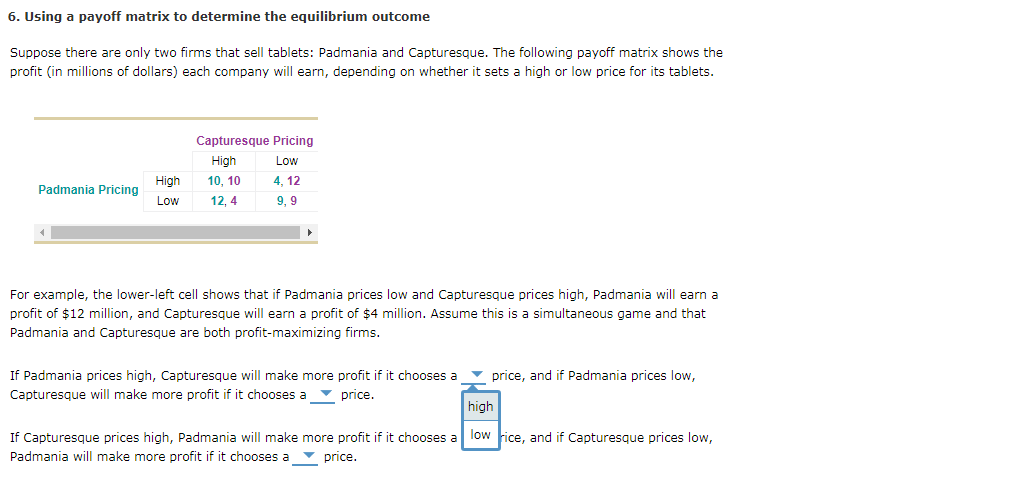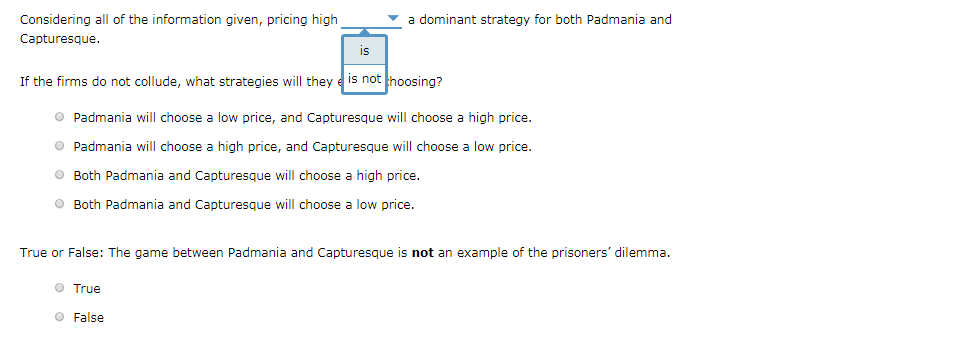### Create an Account

Home / Questions / 6. Using a payoff matrix to determine the equilibrium outcome Suppose there are only two firms that

# 6. Using a payoff matrix to determine the equilibrium outcome Suppose there are only two firms thatSubscribe To Get Solution